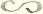# Transcription

## 3-15-40. George Howard Darwin to H. Poincaré

Oct. 22.01

Newnham Grange–Cambridge

Dear Monsieur Poincaré,

Your M.S. has arrived.11Poincaré a annoncé l’envoi de son mémoire par lettre (§ 3-15-39). I shall venture to annotate it a little in red ink with instructions to the printers in English as I think it may save much trouble in the proofs. Of course I have experience of their ways which you cannot have.

I think it might be useful if I append a note in my own name to explain the identities of your $R$’s and my $\mathfrak{P}$ and $\mathbf{P}$. It has taken me nearly half an hour to make it out & I may as well save others the trouble. In doing this however I have discovered two things. I am unable to find any $R_{0}$ in the Acta, but I gather from your M.S. that it must denote $\mathfrak{P}_{0}(v)$ a constant or unity.

Secondly in yr explanation you do not really follow yr notation of the Acta.22In the published memoir, Poincaré notes that he and Darwin do not employ the same notation, and that he employs a notation different from that employed in his Acta paper (1902, 335–336). You write there $R^{(k)}_{n,i}$ and $n$ is clearly the degree of the harmonic and $i$ its order, because you refer to its becoming

 $A(\rho^{2}-e^{2})^{\frac{1}{2}i}D^{i+n}(p^{2}-e^{2})^{n}$

in the case of the spheroid. Hence where in yr M.S. you write $R^{\prime}_{0,2}$ and $R^{\prime}_{0,3}$ you mean $R^{\prime}_{2,0}$ and $R^{\prime}_{3,0}$. Accordingly I propose (with your consent) to correct this.33Darwin’s note was inserted in Poincaré’s paper (Poincaré 1902, 336). I then obtain the following for your new $R$’s.

 $\displaystyle R_{1}$ $\displaystyle=\mathfrak{P}_{0}(v),$ $\displaystyle R_{2}$ $\displaystyle=\mathfrak{P}^{1}_{1}(v),$ $\displaystyle R_{3}$ $\displaystyle=\mathfrak{P}_{2}(v),$ $\displaystyle R_{4}$ $\displaystyle=\mathfrak{P}^{2}_{2}(v),$ $\displaystyle R_{4}$ $\displaystyle=\mathfrak{P}_{3}(v),$

Of course if in any case you dislike the mode of printing which I shall suggest it will be open to you to correct it in proof.

I do not think our printers have any type for yourexcept one like this $\backsim$ which has been used by some writers to denote a difference without regard to sign. On the other hand we have $\propto$ which has been used as equivalent to “varies as” & this is your meaning. I suggest therefore $\propto$ to replace.

Our printers always make such a thing as $\frac{\xi}{2}$ look very ugly, & so I write $\frac{1}{2}\xi$. This moreover saves the compositor much trouble. I have suggested this in many places.

I am afraid the French will be very badly set up & so I will look over a first proof before sending it on to you. I will write again if anything occurs to me. I have not yet had time to master your method. I am besides very busy for some days to come.

Yours sincerely,

G. H. Darwin

ALS 4p. Collection particulière, Paris.

## References

• H. Poincaré (1902) Sur la stabilité de l’équilibre des figures piriformes affectées par une masse fluide en rotation. Philosophical Transactions of the Royal Society A 198, pp. 333–373. External Links: Link

#### Titre (dcterms:title)

George Howard Darwin à Henri Poincaré, 22 octobre 1901

1901-10-22

#### Type (ahpo:documentType)

(fr) Lettre autographe signée

40

#### Droits (ahpo:rightsHolder)

Archives Henri Poincaré

4

en

Gharnati Ph.D.